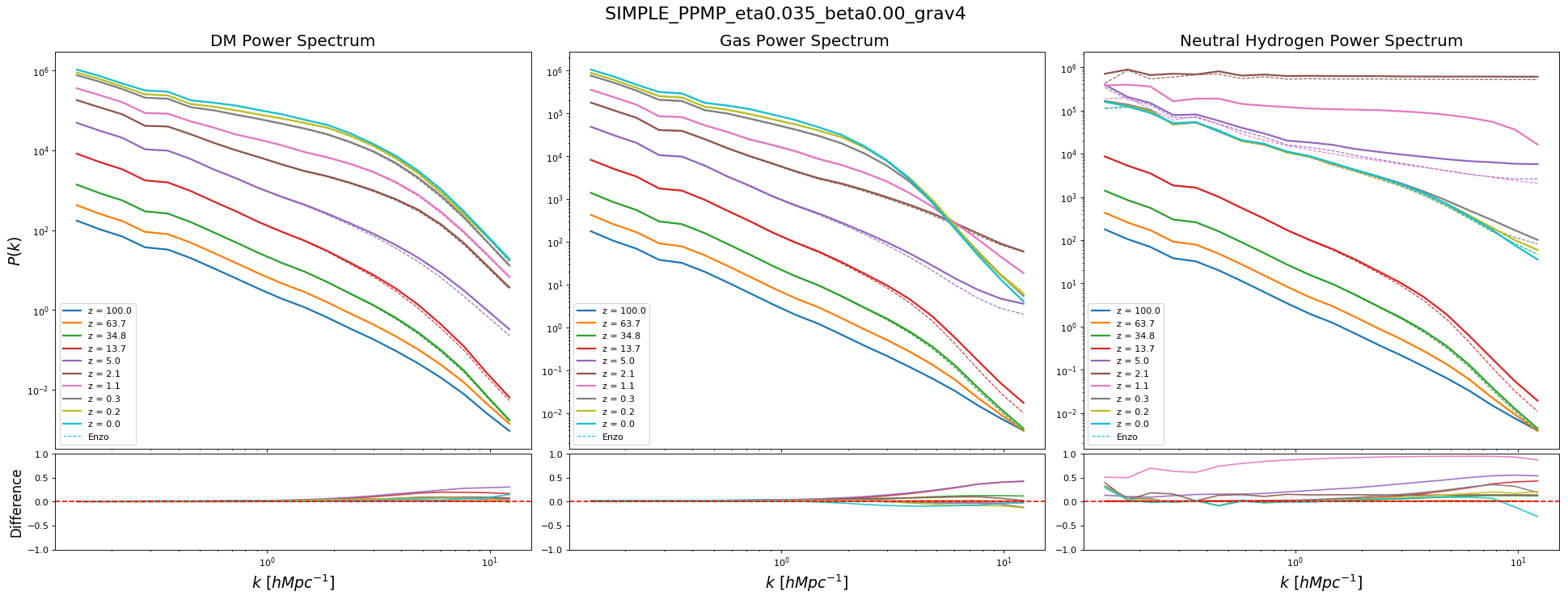Here is the comparison for the average temperature in a cosmological simulation.All codes are using the 4th order scheme for the potential gradient.

Cholla is using PPMP HLLC and SIMPLE, $\beta=0$.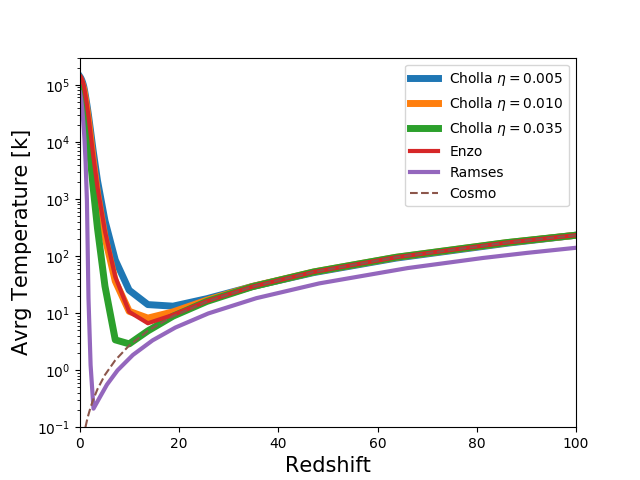Now using Cooling and UV Background: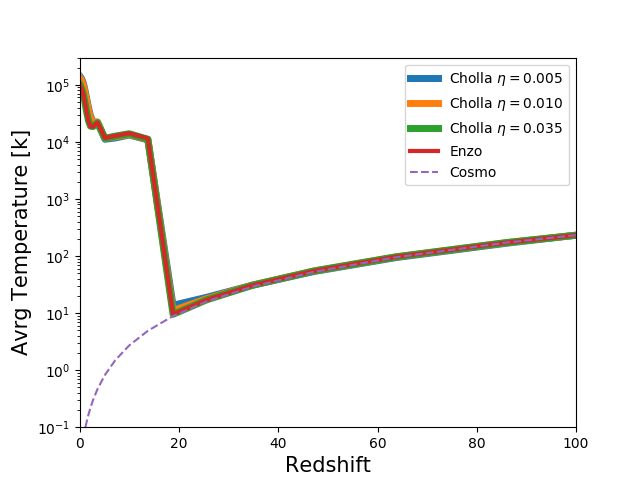## Power Spectrum

Here is the power spectrum for Cholla and Enzo without using Cooling/UV:

### $\eta=0.005$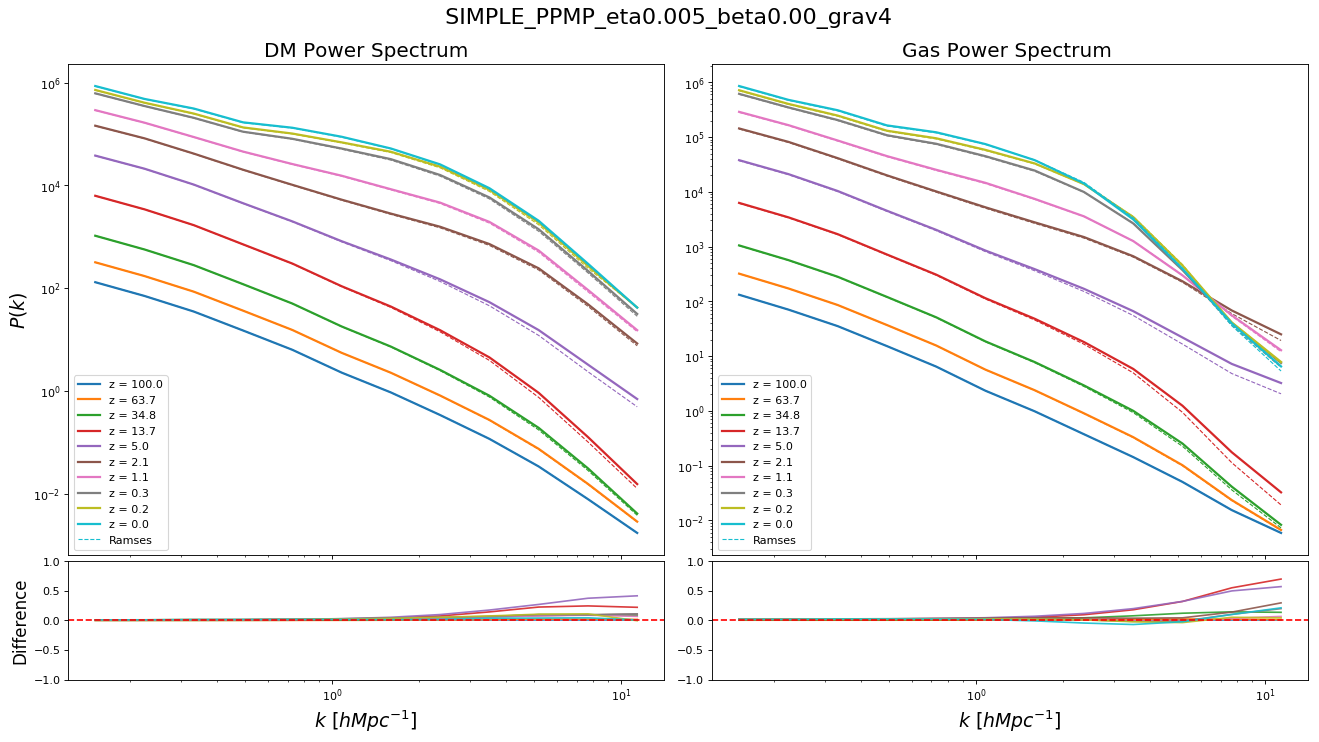### $\eta=0.010$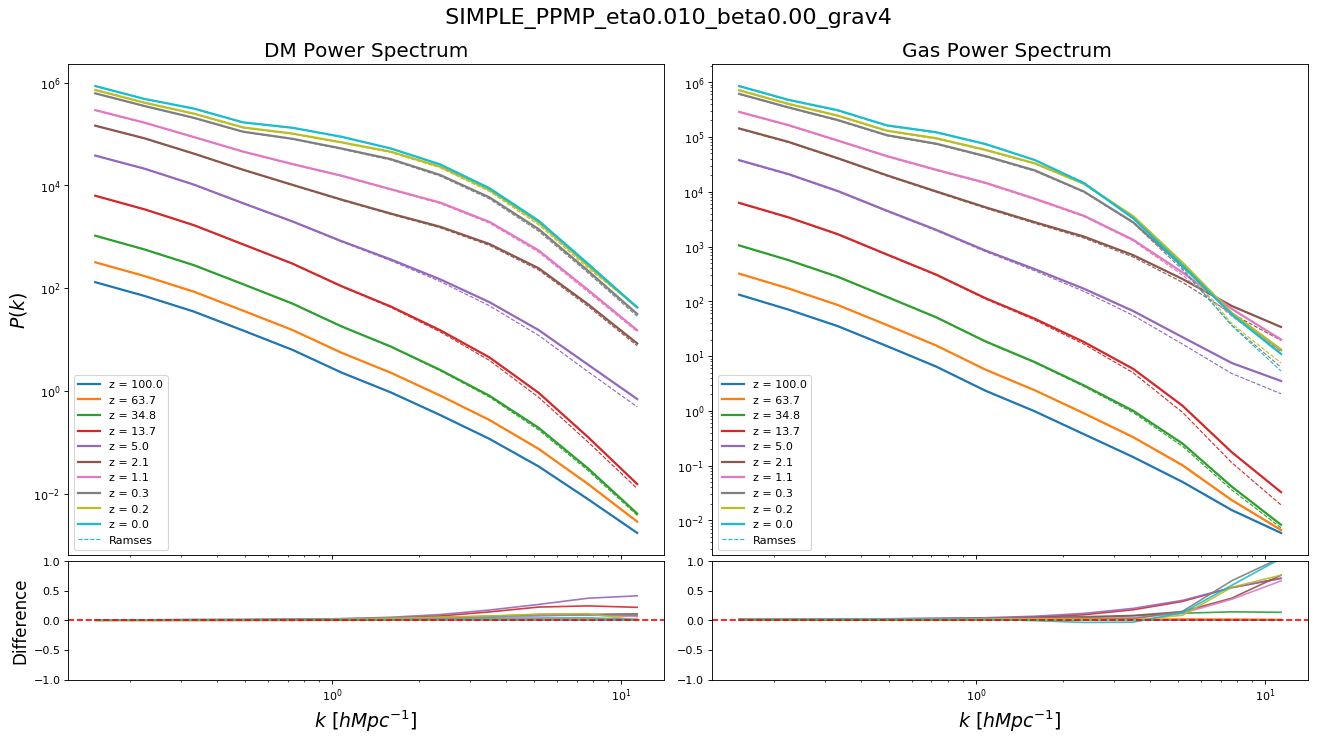### $\eta=0.035$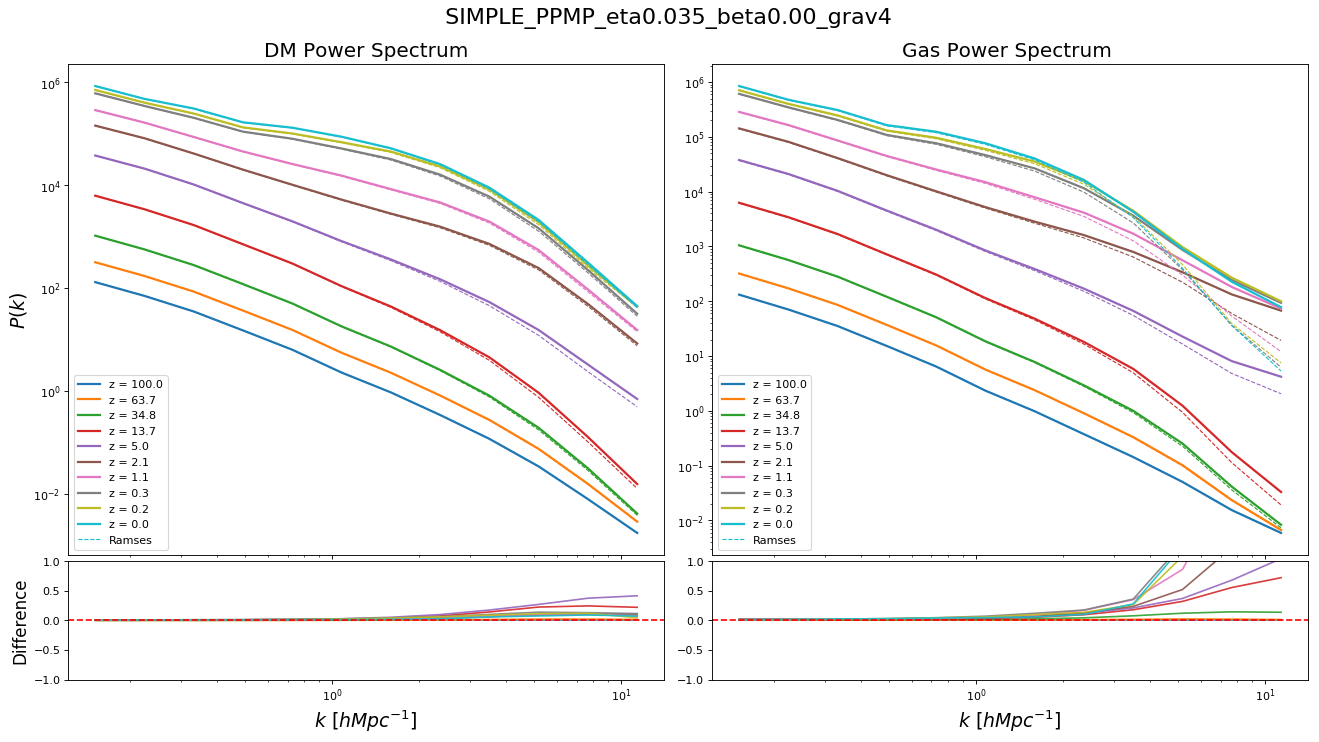### $\eta=0.030$ and $\beta=0.2$:

These where the parameters that best matched Ramses: Gas is much colder.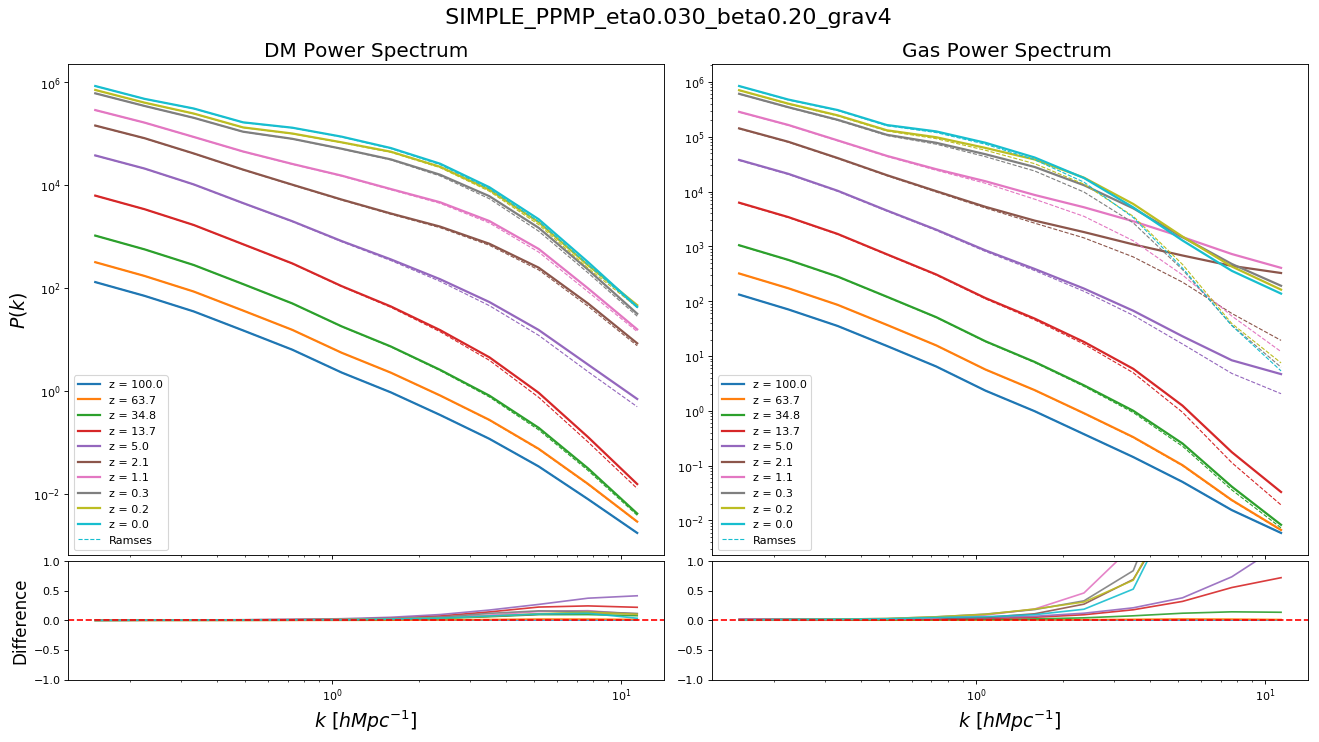Now using Cooling and UV Background:

### $\eta=0.010$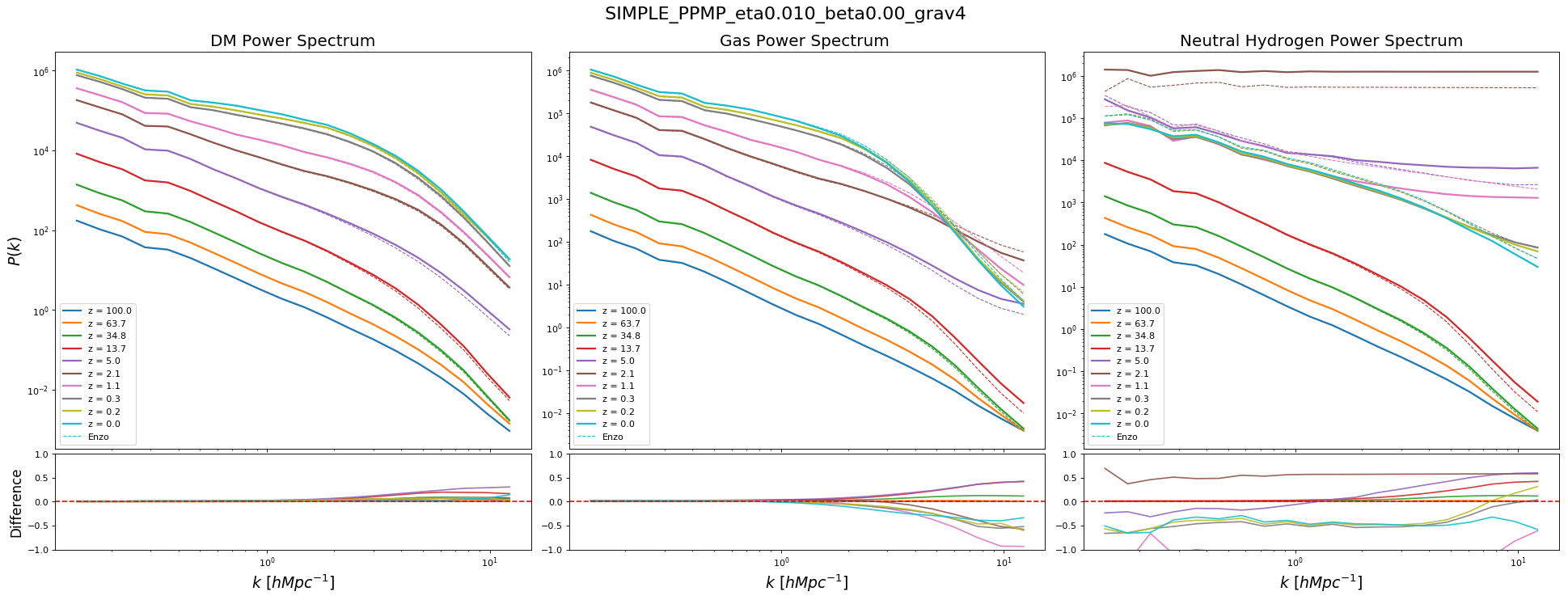### $\eta=0.035$